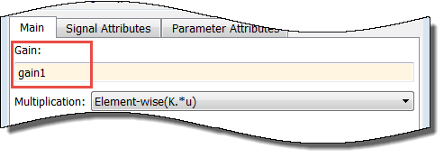## Specify Parameters for Design Exploration

This topic shows how to select parameters of a Simulink® model for design exploration in the Sensitivity Analyzer. After you select the parameters, you generate parameter samples by varying the parameter values in a specific range, and evaluate your design requirements for each combination of parameter values.

### Add Model Parameters as Variables

The software can only evaluate variables that are in use by the model. Create variables in the MATLAB® or model workspace, and specify your Simulink model or block parameters using these variables. If you have already specified model parameters as variables, Select Parameters for Design Exploration.

In this figure, the Gain parameter of a Gain block is specified as a numerical value.To evaluate design requirements using the Gain parameter, specify it as variable `gain1`:

1. Create the variable `gain1` in one of the following ways:

2. Specify the block parameter as variable `gain1` in the Gain block dialog box.You can now select `gain1` for evaluation. See, Select Parameters for Design Exploration.

### Select Parameters for Design Exploration

In the Sensitivity Analyzer, in the Sensitivity Analysis tab, click Select Parameters to open the Select model variables dialog box.

Use this dialog box to select parameters to vary. The table lists the variables that the model uses to set block parameter values. The variables can reside in the model workspace, the base workspace, or a data dictionary.

Select variables by clicking the check box next to each variable. If your model contains many variables, filter the list by typing in the Filter by variable name field. The Used By column lists all blocks in the model that use the variable. When a variable is used in more than one block, all blocks are listed. To highlight blocks in the model that use the variable, click the block name.The variables that you select must have a numeric value that uses the data type `double`. If the value of a variable is not a `double` number, use these techniques:

• To select a single element or a subset of a matrix or array variable, click Specify expression indexing if necessary.Enter an expression such as `myArray(2)`, which selects the second element of an array variable `myArray`.

After you type the expression, press the Enter key to add the variable to the list of model variables.

• To use a variable of a numeric data type other than `double`, convert the variable to a `Simulink.Parameter` object, which separates a parameter value from its data type. Set the `Value` property to a default `double` number, and use the `DataType` property to control the data type.

• To use the value of a `Simulink.Parameter` object, specify the `Value` property. Enter the expression `myParamObj.Value`.

• To use a numeric field of a structure, enter `myStruct.PID.P1`. If you store the structure in a `Simulink.Parameter` object, enter `myStruct.Value.PID.P1`.

• To use one cell of a cell array, enter `myCells{3}`.

You cannot use mathematical expressions such as a + b. Sometimes, models have parameters that are not explicitly defined in the model itself. For example, a gain `k` could be defined in the MATLAB workspace as `k = a + b`, where `a` and `b` are not defined in the model but `k` is used. To add these independent parameters, see Add Model Parameters as Variables.

## Related Topics